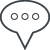# Math, Model, Measure

SystemVision Cloud is the perfect online circuit simulation environment for engineering studies.   The cloud-based simulator allows students to explore and verify electronic designs, prior to building a prototype, to both learn more effectively and to be more efficient with time and resources in the lab. It's the perfect modeling tool to bridge the gap between the theoretical math world of transfer functions and physics, and the empirical world of lab measurements.

# Common Emitter Amplifier Circuit

This example was provided by an MSEE student.  The student used SystemVision Cloud in a homework/lab project for an Analog Communications Course. In this lab, the students were asked to design a common emitter amplifier circuit, using SystemVision Cloud, and then verify the functionality by building a physical prototype and measuring the performance.

While exploring various options for the amplifier design, the student used design equations, derived analytically, to determine the relationship between parameter values and the amplifier input-to-output voltage gain. Then, SystemVision Cloud was used to fine-tune the values to achieve and/or verify the desired gain.  The student then built a prototype by soldering the selected parts onto a single-sided, copper-plated board and measuring the result with test equipment.

## Common Emitter Configuration

Figure 1. The Common Emitter Amplifier Circuit

## Theoretical Considerations

Design Specifications: Design a common emitter amplifier to given a give a gain of 15 (23.5 dB) when the source resistance is equal to zero and load resistance is 50 ohms. There is a single power supply value that is chosen between 5V and 10V. Design and simulate the circuit to get a gain. The capacitor values should be 0.1 microfarads.

A few simplifying assumptions and a bit of circuit analysis, followed by simple algebra yields an equation for the amplifier voltage gain as a function of the circuit parameters and a couple of constants:

where  is the so-called p-n junction thermal voltage (~25 mV) and  is the approximate voltage drop for a forward biased p-n junction (0.7 V).

So, for the following values:

This is equivalent to 24.4 dB gain with 180º phase shift.

This result matches favorably with the simulation shown above in Figure 1.

##Figure 2. Common Emitter Prototype Board

Measurements were made on the circuit board in Figure 2.  The input voltage, Vin, was a 10 mV (peak-to-peak) sine wave at both 5 MHz and 10 MHz, supplied by a function generator.  The output voltage, Vout, was measured with an oscilloscope.  At 5 MHz, the output was 120 mV, peak-to-peak, and at 10 MHz it was 122 mV.  This corresponds to a gain of 12 (21.6 dB) and 12.2 (21.7 dB), respectively.

From the simulation, in Figure 1, the 5 MHz value compares relatively well at approximately 19.6 dB.  But the 10 MHz simulation falls off to about 15 dB, rather than 21.7 dB in the measurement.

## Conclusions

Overall, the design equation approximation for the amplifier gain was accurate enough to be useful in selecting component values.  The SystemVision circuit simulation provided a higher degree of accuracy to determine the theoretical behavior of the amplifier, over the frequency range of interest.  The measured results agreed within reasonable experimental error.

# Resources for further reading

Wikipedia Common Emitter Amplifiers

Electronics Tutorials - Common Emitter Amplifiers

All About Circuits - Common Emitter Amplifiers

# AcknowledgmentsThanks to Ramya Mahadevan for contributing the circuits and photos for this blog. Ramya is an MSEE student at the University of Illinois, Chicago.

##Comments

Please log in to comment.